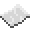# term.getTextColour

Returns the numerical value of the current text colour of the terminal object.ExampleGet text colour
Prints the numerical value of the current term's text colour.
Code
```<nowiki>
print(term.getTextColour())
</nowiki>
```
Output
```1
```ExampleGet text colour name
Gets the current term's text colour, and then matches it against a lookup table to get its name.
Code
```<nowiki>
local lookup = {
 = "white",  = "orange",  = "magenta",  = "lightBlue",
 = "yellow",  = "lime",  = "pink",  = "gray",
 = "lightGray",   = "cyan",  = "purple",  = "blue",
 = "brown",   = "green",  = "red",  = "black"
}
local colour = term.getTextColour()
print(lookup[colour])
</nowiki>
```
Output
```white
```

term.getTextColour
Function
Syntax
term.getTextColour()
Returns number colour
API term
Source CC:Tweaked (source)